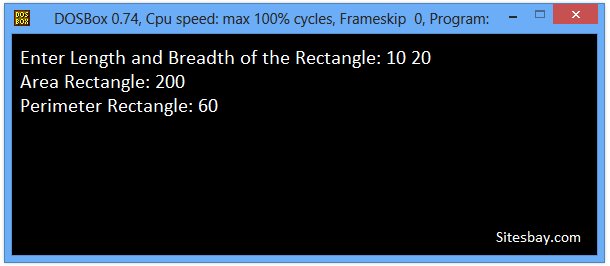# Calculate Area and Perimeter of Rectangle in C++

## C++ Program to Calculate Area and Perimeter of Rectangle

To calculate area and perimeter of a square and rectangle we need length and breadth of the rectangle. First we receiver two variable from user using Cin>> function. and print output on screen using cout>> function

To understand below example, you have must knowledge of following C++ programming topics; Cin and Cout Function in C++ Here Cout<< Function in C++ are used for print output on screen and Cin>> function in C++ are used for get input from user.

## C++ Program to Calculate Area and Perimeter of Rectangle

```#include<iostream.h>
#include<conio.h>

void main()
{
clrscr();
int len, bre, peri, area;
cout<<"Enter Length and Breadth of the Rectangle: ";
cin>>len>>bre;
area=len*bre;
peri=(2*len)+(2*bre);
cout<<"Area of Rectangle: "<<area<<"\tPerimeter Rectangle: "<<peri;
getch();
}
```

## Output

```Enter Length and Breadth of the Rectangle: 10 20
Area Rectangle: 200
Perimeter Rectangle: 60
```## Pure VPN Privide Lowest Price VPN Just @ \$1.65. Per Month with Non Detected IP Lowest Price Non Detected IP VPN